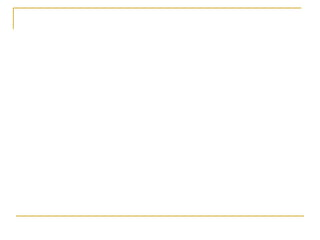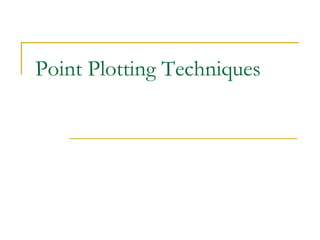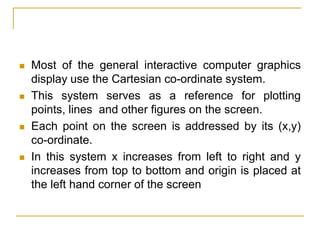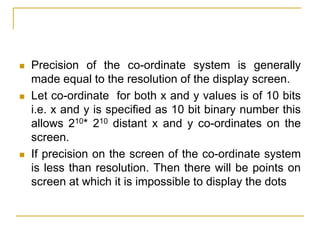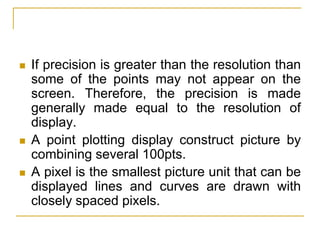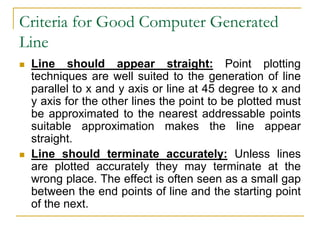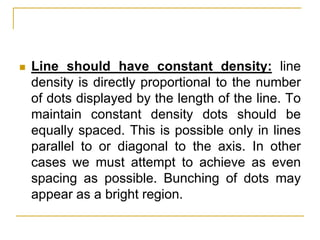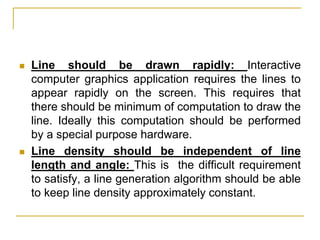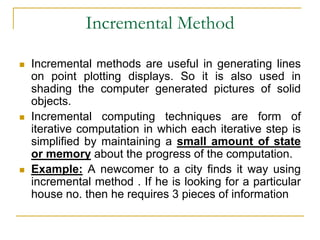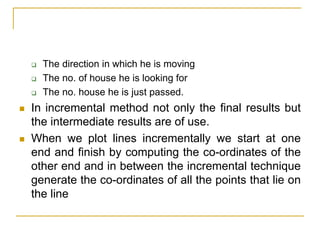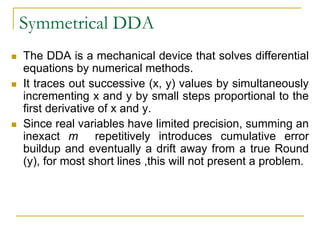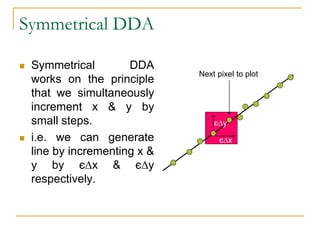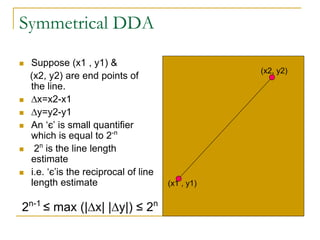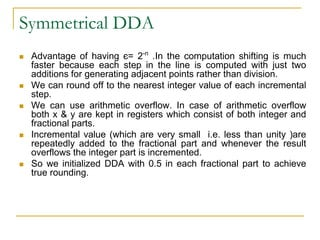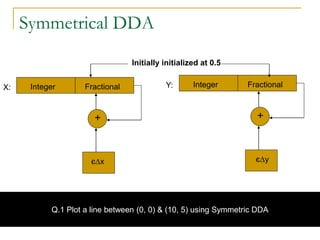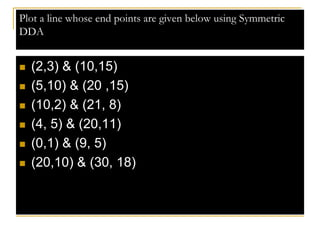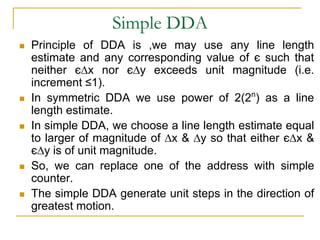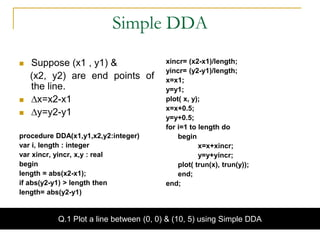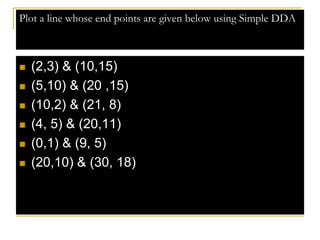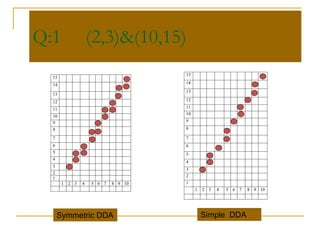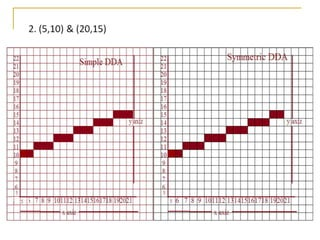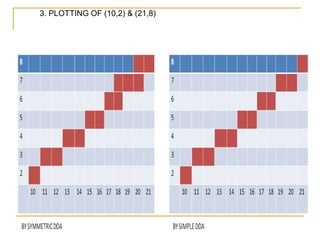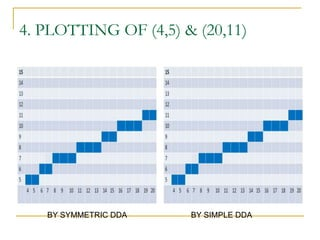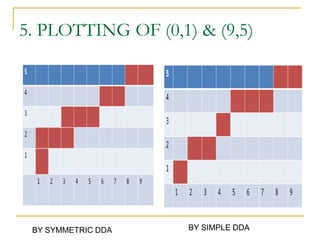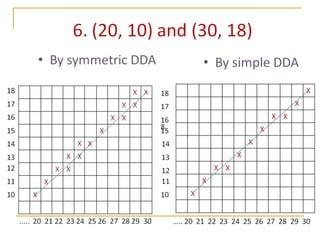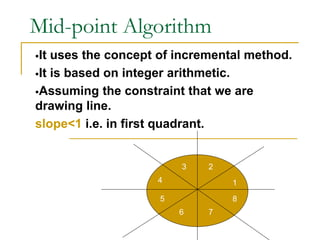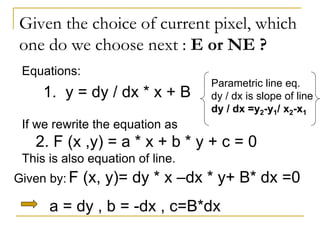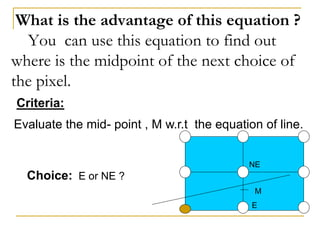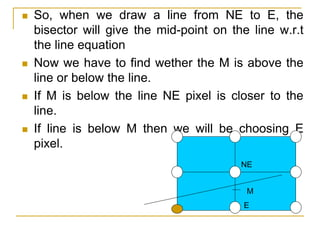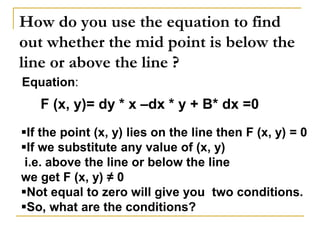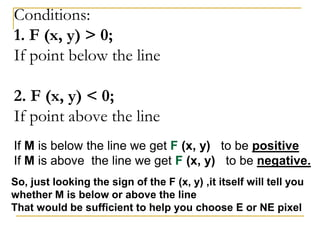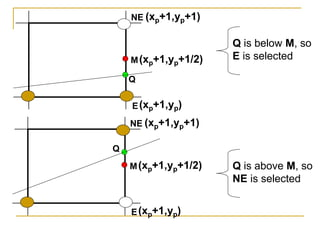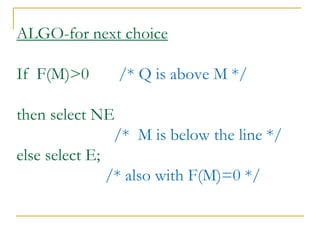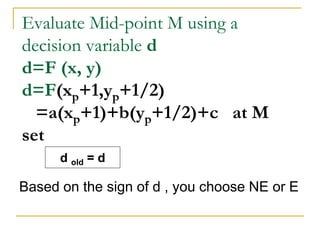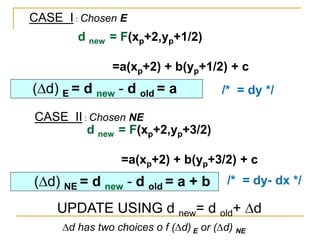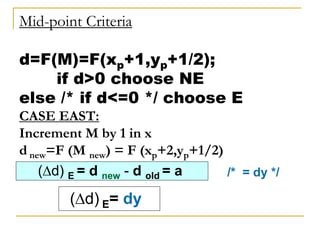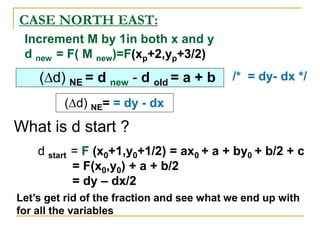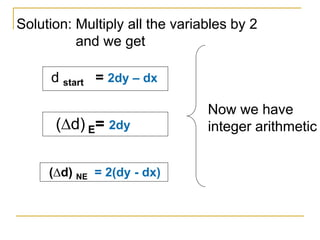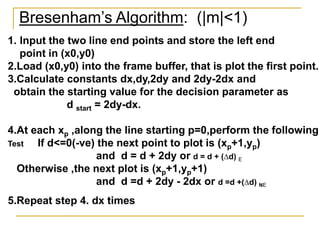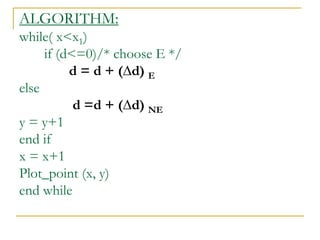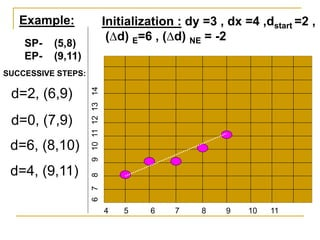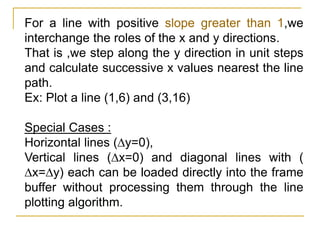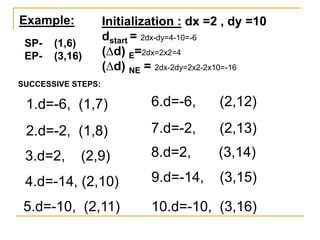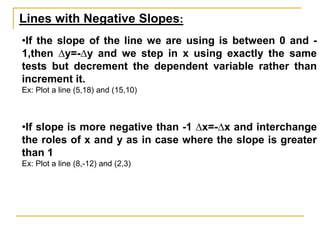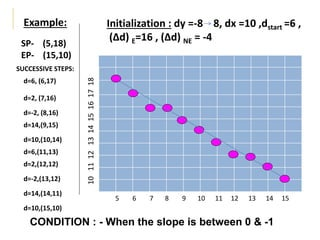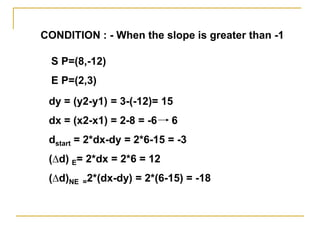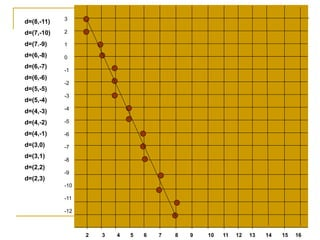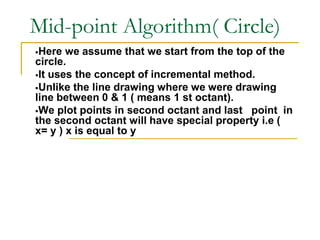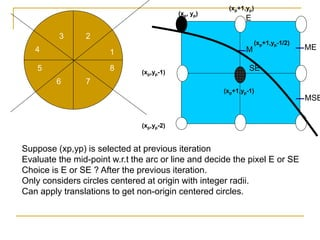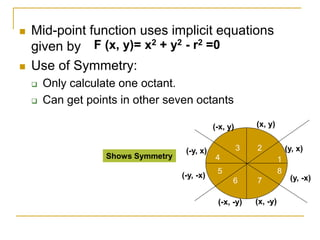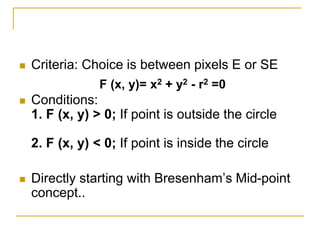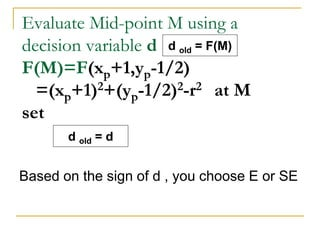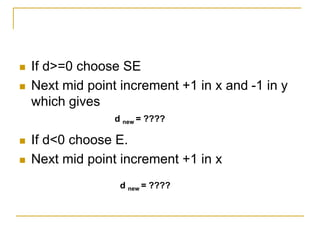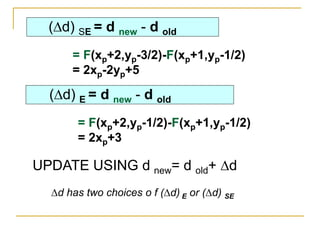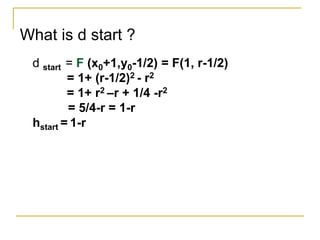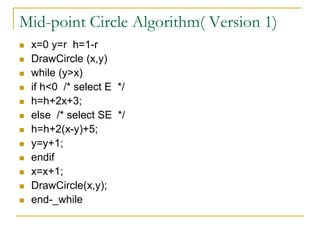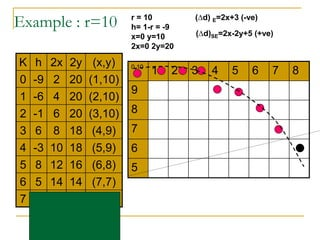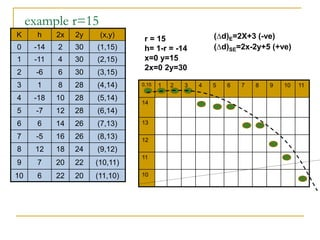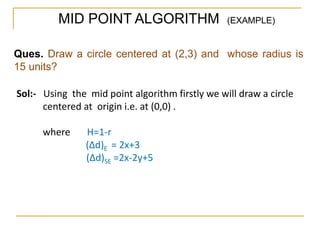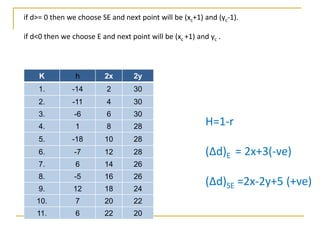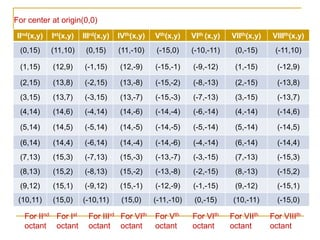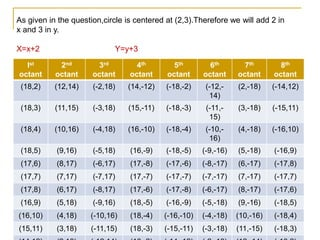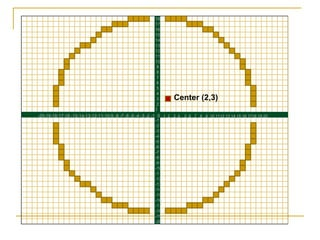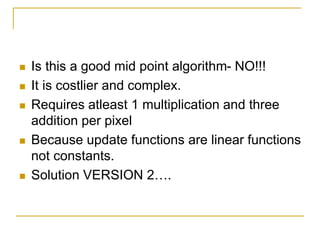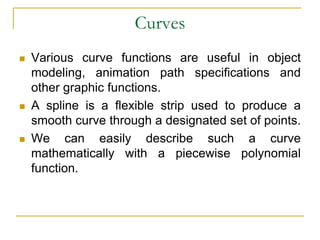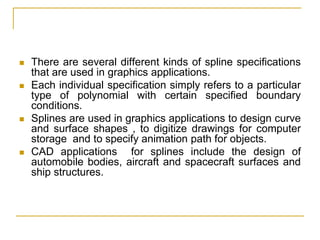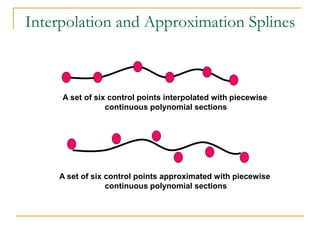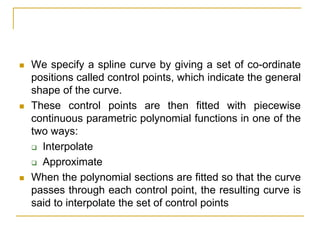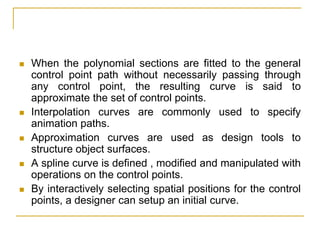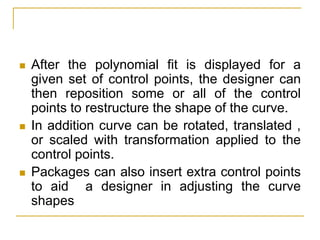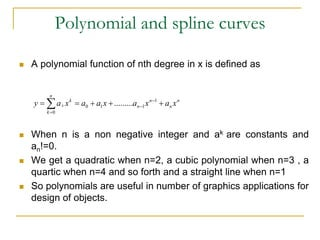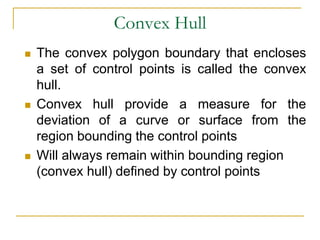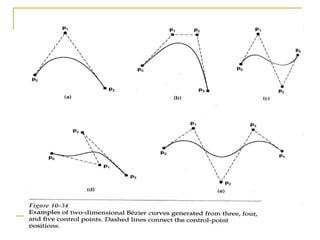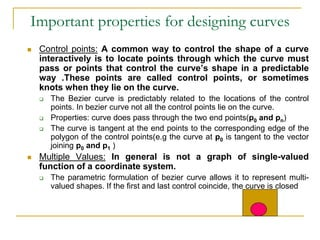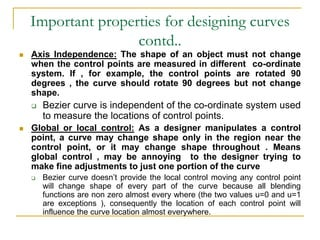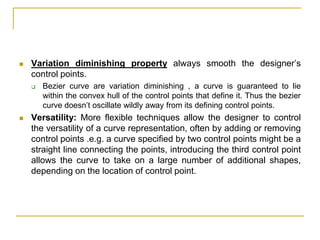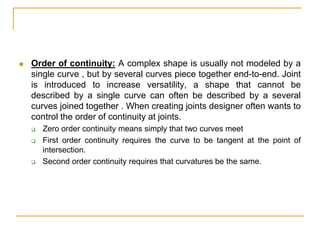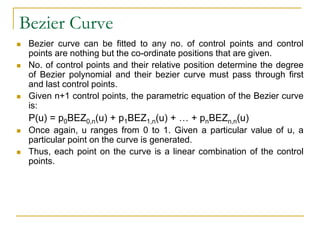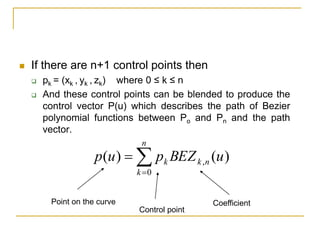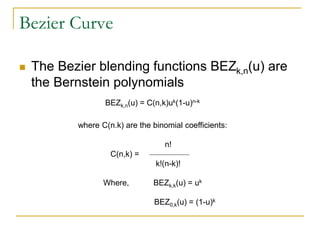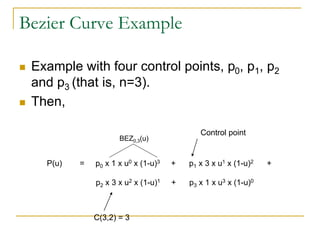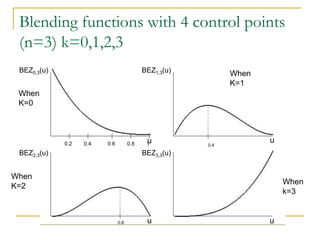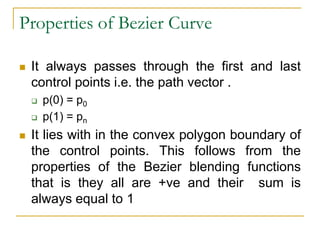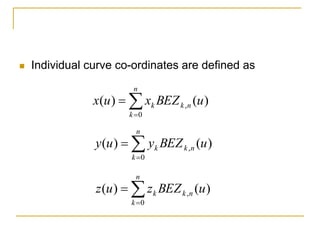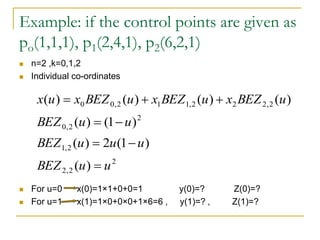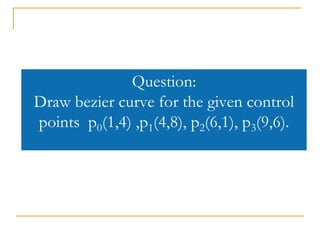1 de 86

### 99995320.ppt

1. Point Plotting Techniques
2.  Most of the general interactive computer graphics display use the Cartesian co-ordinate system.  This system serves as a reference for plotting points, lines and other figures on the screen.  Each point on the screen is addressed by its (x,y) co-ordinate.  In this system x increases from left to right and y increases from top to bottom and origin is placed at the left hand corner of the screen
3.  Precision of the co-ordinate system is generally made equal to the resolution of the display screen.  Let co-ordinate for both x and y values is of 10 bits i.e. x and y is specified as 10 bit binary number this allows 210* 210 distant x and y co-ordinates on the screen.  If precision on the screen of the co-ordinate system is less than resolution. Then there will be points on screen at which it is impossible to display the dots
4.  If precision is greater than the resolution than some of the points may not appear on the screen. Therefore, the precision is made generally made equal to the resolution of display.  A point plotting display construct picture by combining several 100pts.  A pixel is the smallest picture unit that can be displayed lines and curves are drawn with closely spaced pixels.
5. Criteria for Good Computer Generated Line  Line should appear straight: Point plotting techniques are well suited to the generation of line parallel to x and y axis or line at 45 degree to x and y axis for the other lines the point to be plotted must be approximated to the nearest addressable points suitable approximation makes the line appear straight.  Line should terminate accurately: Unless lines are plotted accurately they may terminate at the wrong place. The effect is often seen as a small gap between the end points of line and the starting point of the next.
6.  Line should have constant density: line density is directly proportional to the number of dots displayed by the length of the line. To maintain constant density dots should be equally spaced. This is possible only in lines parallel to or diagonal to the axis. In other cases we must attempt to achieve as even spacing as possible. Bunching of dots may appear as a bright region.
7.  Line should be drawn rapidly: Interactive computer graphics application requires the lines to appear rapidly on the screen. This requires that there should be minimum of computation to draw the line. Ideally this computation should be performed by a special purpose hardware.  Line density should be independent of line length and angle: This is the difficult requirement to satisfy, a line generation algorithm should be able to keep line density approximately constant.
8. Incremental Method  Incremental methods are useful in generating lines on point plotting displays. So it is also used in shading the computer generated pictures of solid objects.  Incremental computing techniques are form of iterative computation in which each iterative step is simplified by maintaining a small amount of state or memory about the progress of the computation.  Example: A newcomer to a city finds it way using incremental method . If he is looking for a particular house no. then he requires 3 pieces of information
9.  The direction in which he is moving  The no. of house he is looking for  The no. house he is just passed.  In incremental method not only the final results but the intermediate results are of use.  When we plot lines incrementally we start at one end and finish by computing the co-ordinates of the other end and in between the incremental technique generate the co-ordinates of all the points that lie on the line
10. Symmetrical DDA  The DDA is a mechanical device that solves differential equations by numerical methods.  It traces out successive (x, y) values by simultaneously incrementing x and y by small steps proportional to the first derivative of x and y.  Since real variables have limited precision, summing an inexact m repetitively introduces cumulative error buildup and eventually a drift away from a true Round (y), for most short lines ,this will not present a problem.
11. Symmetrical DDA  Symmetrical DDA works on the principle that we simultaneously increment x & y by small steps.  i.e. we can generate line by incrementing x & y by є∆x & є∆y respectively. є∆x є∆y Next pixel to plot
12. Symmetrical DDA  Suppose (x1 , y1) & (x2, y2) are end points of the line.  ∆x=x2-x1  ∆y=y2-y1  An ‘є’ is small quantifier which is equal to 2-n  2n is the line length estimate  i.e. ‘є’is the reciprocal of line length estimate 2n-1 ≤ max (|∆x| |∆y|) ≤ 2n (x1 , y1) (x2, y2)
13. Symmetrical DDA  Advantage of having є= 2-n .In the computation shifting is much faster because each step in the line is computed with just two additions for generating adjacent points rather than division.  We can round off to the nearest integer value of each incremental step.  We can use arithmetic overflow. In case of arithmetic overflow both x & y are kept in registers which consist of both integer and fractional parts.  Incremental value (which are very small i.e. less than unity )are repeatedly added to the fractional part and whenever the result overflows the integer part is incremented.  So we initialized DDA with 0.5 in each fractional part to achieve true rounding.
14. Symmetrical DDA X: Integer Fractional + є∆x Y: Integer Fractional + є∆y Initially initialized at 0.5 Q.1 Plot a line between (0, 0) & (10, 5) using Symmetric DDA
15. Plot a line whose end points are given below using Symmetric DDA  (2,3) & (10,15)  (5,10) & (20 ,15)  (10,2) & (21, 8)  (4, 5) & (20,11)  (0,1) & (9, 5)  (20,10) & (30, 18)
16. Simple DDA  Principle of DDA is ,we may use any line length estimate and any corresponding value of є such that neither є∆x nor є∆y exceeds unit magnitude (i.e. increment ≤1).  In symmetric DDA we use power of 2(2n ) as a line length estimate.  In simple DDA, we choose a line length estimate equal to larger of magnitude of ∆x & ∆y so that either є∆x & є∆y is of unit magnitude.  So, we can replace one of the address with simple counter.  The simple DDA generate unit steps in the direction of greatest motion.
17. Simple DDA  Suppose (x1 , y1) & (x2, y2) are end points of the line.  ∆x=x2-x1  ∆y=y2-y1 procedure DDA(x1,y1,x2,y2:integer) var i, length : integer var xincr, yincr, x,y : real begin length = abs(x2-x1); if abs(y2-y1) > length then length= abs(y2-y1) xincr= (x2-x1)/length; yincr= (y2-y1)/length; x=x1; y=y1; plot( x, y); x=x+0.5; y=y+0.5; for i=1 to length do begin x=x+xincr; y=y+yincr; plot( trun(x), trun(y)); end; end; Q.1 Plot a line between (0, 0) & (10, 5) using Simple DDA
18. Plot a line whose end points are given below using Simple DDA  (2,3) & (10,15)  (5,10) & (20 ,15)  (10,2) & (21, 8)  (4, 5) & (20,11)  (0,1) & (9, 5)  (20,10) & (30, 18)
19. Q:1 (2,3)&(10,15) 15 14 13 12 11 10 9 8 7 6 5 4 3 2 1 1 2 3 4 5 6 7 8 9 10 15 14 13 12 11 10 9 8 7 6 5 4 3 2 1 1 2 3 4 5 6 7 8 9 10 Symmetric DDA Simple DDA
20. 3. PLOTTING OF (10,2) & (21,8)
21. 4. PLOTTING OF (4,5) & (20,11) BY SYMMETRIC DDA BY SIMPLE DDA
22. 5. PLOTTING OF (0,1) & (9,5) BY SYMMETRIC DDA BY SIMPLE DDA
23. Mid-point Algorithm It uses the concept of incremental method. It is based on integer arithmetic. Assuming the constraint that we are drawing line. slope<1 i.e. in first quadrant. 1 1 1 2 3 4 5 6 7 8
24. Given the choice of current pixel, which one do we choose next : E or NE ? Equations: 1. y = dy / dx * x + B If we rewrite the equation as 2. F (x ,y) = a * x + b * y + c = 0 This is also equation of line. Given by: F (x, y)= dy * x –dx * y+ B* dx =0 a = dy , b = -dx , c=B*dx Parametric line eq. dy / dx is slope of line dy / dx =y2-y1/ x2-x1
25. What is the advantage of this equation ? You can use this equation to find out where is the midpoint of the next choice of the pixel. Criteria: Evaluate the mid- point , M w.r.t the equation of line. Choice: E or NE ? M NE E
26.  So, when we draw a line from NE to E, the bisector will give the mid-point on the line w.r.t the line equation  Now we have to find wether the M is above the line or below the line.  If M is below the line NE pixel is closer to the line.  If line is below M then we will be choosing E pixel. M NE E
27. How do you use the equation to find out whether the mid point is below the line or above the line ? Equation: F (x, y)= dy * x –dx * y + B* dx =0 If the point (x, y) lies on the line then F (x, y) = 0 If we substitute any value of (x, y) i.e. above the line or below the line we get F (x, y) ≠ 0 Not equal to zero will give you two conditions. So, what are the conditions?
28. Conditions: 1. F (x, y) > 0; If point below the line 2. F (x, y) < 0; If point above the line If M is below the line we get F (x, y) to be positive If M is above the line we get F (x, y) to be negative. So, just looking the sign of the F (x, y) ,it itself will tell you whether M is below or above the line That would be sufficient to help you choose E or NE pixel
29. M Q NE E (xp+1,yp+1) (xp+1,yp+1/2) (xp+1,yp) M Q NE E (xp+1,yp+1) (xp+1,yp+1/2) (xp+1,yp) Q is below M, so E is selected Q is above M, so NE is selected
30. ALGO-for next choice If F(M)>0 /* Q is above M */ then select NE /* M is below the line */ else select E; /* also with F(M)=0 */
31. Evaluate Mid-point M using a decision variable d d=F (x, y) d=F(xp+1,yp+1/2) =a(xp+1)+b(yp+1/2)+c at M set d old = d Based on the sign of d , you choose NE or E
32. CASE I : Chosen E d new = F(xp+2,yp+1/2) =a(xp+2) + b(yp+1/2) + c (∆d) E = d new - d old = a /* = dy */ CASE II : Chosen NE d new = F(xp+2,yp+3/2) =a(xp+2) + b(yp+3/2) + c (∆d) NE = d new - d old = a + b /* = dy- dx */ UPDATE USING d new= d old+ ∆d ∆d has two choices o f (∆d) E or (∆d) NE
33. Mid-point Criteria d=F(M)=F(xp+1,yp+1/2); if d>0 choose NE else /* if d<=0 */ choose E CASE EAST: Increment M by 1 in x d new=F (M new) = F (xp+2,yp+1/2) (∆d) E = d new - d old = a /* = dy */ (∆d) E= dy
34. CASE NORTH EAST: Increment M by 1in both x and y d new = F( M new)=F(xp+2,yp+3/2) (∆d) NE = d new - d old = a + b /* = dy- dx */ (∆d) NE= = dy - dx What is d start ? d start = F (x0+1,y0+1/2) = ax0 + a + by0 + b/2 + c = F(x0,y0) + a + b/2 = dy – dx/2 Let’s get rid of the fraction and see what we end up with for all the variables
35. Solution: Multiply all the variables by 2 and we get d start = 2dy – dx (∆d) NE = 2(dy - dx) (∆d) E= 2dy Now we have integer arithmetic
36. Bresenham’s Algorithm: (|m|<1) 1. Input the two line end points and store the left end point in (x0,y0) 2.Load (x0,y0) into the frame buffer, that is plot the first point. 3.Calculate constants dx,dy,2dy and 2dy-2dx and obtain the starting value for the decision parameter as d start = 2dy-dx. 4.At each xp ,along the line starting p=0,perform the following Test If d<=0(-ve) the next point to plot is (xp+1,yp) and d = d + 2dy or d = d + (∆d) E Otherwise ,the next plot is (xp+1,yp+1) and d =d + 2dy - 2dx or d =d +(∆d) NE 5.Repeat step 4. dx times
37. ALGORITHM: while( x<x1) if (d<=0)/* choose E */ d = d + (∆d) E else d =d + (∆d) NE y = y+1 end if x = x+1 Plot_point (x, y) end while
38. Example: SP- (5,8) EP- (9,11) SUCCESSIVE STEPS: Initialization : dy =3 , dx =4 ,dstart =2 , (∆d) E=6 , (∆d) NE = -2 4 5 6 7 8 9 10 11 6 7 8 9 10 11 12 13 14 d=2, (6,9) d=0, (7,9) d=6, (8,10) d=4, (9,11)
39. For a line with positive slope greater than 1,we interchange the roles of the x and y directions. That is ,we step along the y direction in unit steps and calculate successive x values nearest the line path. Ex: Plot a line (1,6) and (3,16) Special Cases : Horizontal lines (∆y=0), Vertical lines (∆x=0) and diagonal lines with ( ∆x=∆y) each can be loaded directly into the frame buffer without processing them through the line plotting algorithm.
40. Example: SP- (1,6) EP- (3,16) SUCCESSIVE STEPS: Initialization : dx =2 , dy =10 dstart = 2dx-dy=4-10=-6 (∆d) E=2dx=2x2=4 (∆d) NE = 2dx-2dy=2x2-2x10=-16 1.d=-6, (1,7) 2.d=-2, (1,8) 3.d=2, (2,9) 4.d=-14, (2,10) 5.d=-10, (2,11) 6.d=-6, (2,12) 7.d=-2, (2,13) 8.d=2, (3,14) 9.d=-14, (3,15) 10.d=-10, (3,16)
41. Lines with Negative Slopes: •If the slope of the line we are using is between 0 and - 1,then ∆y=-∆y and we step in x using exactly the same tests but decrement the dependent variable rather than increment it. Ex: Plot a line (5,18) and (15,10) •If slope is more negative than -1 ∆x=-∆x and interchange the roles of x and y as in case where the slope is greater than 1 Ex: Plot a line (8,-12) and (2,3)
42. Example: SP- (5,18) EP- (15,10) SUCCESSIVE STEPS: Initialization : dy =-8 8, dx =10 ,dstart =6 , (∆d) E=16 , (∆d) NE = -4 5 6 7 8 9 10 11 12 13 14 15 10 11 12 13 14 15 16 17 18 d=6, (6,17) d=2, (7,16) d=-2, (8,16) d=14,(9,15) d=10,(10,14) d=6,(11,13) d=2,(12,12) d=-2,(13,12) d=14,(14,11) d=10,(15,10) CONDITION : - When the slope is between 0 & -1
43. CONDITION : - When the slope is greater than -1 S P=(8,-12) E P=(2,3) dy = (y2-y1) = 3-(-12)= 15 dx = (x2-x1) = 2-8 = -6 6 dstart = 2*dx-dy = 2*6-15 = -3 (∆d) E= 2*dx = 2*6 = 12 (∆d)NE =2*(dx-dy) = 2*(6-15) = -18
44. d=(8,-11) d=(7,-10) d=(7.-9) d=(6,-8) d=(6,-7) d=(6,-6) d=(5,-5) d=(5,-4) d=(4,-3) d=(4,-2) d=(4,-1) d=(3,0) d=(3,1) d=(2,2) d=(2,3) 2 3 4 5 6 7 8 9 10 11 12 13 14 15 16 3 2 1 0 -1 -2 -3 -4 -5 -6 -7 -8 -9 -10 -11 -12
45. Mid-point Algorithm( Circle) Here we assume that we start from the top of the circle. It uses the concept of incremental method. Unlike the line drawing where we were drawing line between 0 & 1 ( means 1 st octant). We plot points in second octant and last point in the second octant will have special property i.e ( x= y ) x is equal to y 1 1
46. 1 2 3 4 5 6 7 8 (xp,yp-1) (xp,yp-2) (xp+1,yp) M E SE ME MSE (xp, yp) (xp+1,yp-1) (xp+1,yp-1/2) Suppose (xp,yp) is selected at previous iteration Evaluate the mid-point w.r.t the arc or line and decide the pixel E or SE Choice is E or SE ? After the previous iteration. Only considers circles centered at origin with integer radii. Can apply translations to get non-origin centered circles.
47.  Mid-point function uses implicit equations given by  Use of Symmetry:  Only calculate one octant.  Can get points in other seven octants F (x, y)= x2 + y2 - r2 =0 1 2 3 4 5 6 7 8 (x, y) (y, x) (y, -x) (-y, x) (-y, -x) (-x, y) (-x, -y) (x, -y) Shows Symmetry
48.  Criteria: Choice is between pixels E or SE  Conditions: 1. F (x, y) > 0; If point is outside the circle 2. F (x, y) < 0; If point is inside the circle  Directly starting with Bresenham’s Mid-point concept.. F (x, y)= x2 + y2 - r2 =0
49. Evaluate Mid-point M using a decision variable d F(M)=F(xp+1,yp-1/2) =(xp+1)2+(yp-1/2)2-r2 at M set d old = d Based on the sign of d , you choose E or SE d old = F(M)
50.  If d>=0 choose SE  Next mid point increment +1 in x and -1 in y which gives  If d<0 choose E.  Next mid point increment +1 in x d new = ???? d new = ????
51. (∆d) SE = d new - d old = F(xp+2,yp-3/2)-F(xp+1,yp-1/2) = 2xp-2yp+5 (∆d) E = d new - d old UPDATE USING d new= d old+ ∆d ∆d has two choices o f (∆d) E or (∆d) SE = F(xp+2,yp-1/2)-F(xp+1,yp-1/2) = 2xp+3
52. What is d start ? d start = F (x0+1,y0-1/2) = F(1, r-1/2) = 1+ (r-1/2)2 - r2 = 1+ r2 –r + 1/4 -r2 = 5/4-r = 1-r hstart = 1-r
53. Mid-point Circle Algorithm( Version 1)  x=0 y=r h=1-r  DrawCircle (x,y)  while (y>x)  if h<0 /* select E */  h=h+2x+3;  else /* select SE */  h=h+2(x-y)+5;  y=y+1;  endif  x=x+1;  DrawCircle(x,y);  end-_while
54. Example : r=10 r = 10 h= 1-r = -9 x=0 y=10 2x=0 2y=20 (∆d) E=2x+3 (-ve) (∆d)SE=2x-2y+5 (+ve) K h 2x 2y (x,y) 0 -9 2 20 (1,10) 1 -6 4 20 (2,10) 2 -1 6 20 (3,10) 3 6 8 18 (4,9) 4 -3 10 18 (5,9) 5 8 12 16 (6,8) 6 5 14 14 (7,7) 7 6 0,10 1 2 3 4 5 6 7 8 9 8 7 6 5
55. example r=15 r = 15 h= 1-r = -14 x=0 y=15 2x=0 2y=30 (∆d)SE=2x-2y+5 (+ve) K h 2x 2y (x,y) 0 -14 2 30 (1,15) 1 -11 4 30 (2,15) 2 -6 6 30 (3,15) 3 1 8 28 (4,14) 4 -18 10 28 (5,14) 5 -7 12 28 (6,14) 6 6 14 26 (7,13) 7 -5 16 26 (8,13) 8 12 18 24 (9,12) 9 7 20 22 (10,11) 10 6 22 20 (11,10) 0,15 1 2 3 4 5 6 7 8 9 10 11 14 13 12 11 10 (∆d)E=2X+3 (-ve)
56. Ques. Draw a circle centered at (2,3) and whose radius is 15 units? Sol:- Using the mid point algorithm firstly we will draw a circle centered at origin i.e. at (0,0) . where H=1-r (∆d)E = 2x+3 (∆d)SE =2x-2y+5 MID POINT ALGORITHM (EXAMPLE)
57. K h 2x 2y 1. -14 2 30 2. -11 4 30 3. -6 6 30 4. 1 8 28 5. -18 10 28 6. -7 12 28 7. 6 14 26 8. -5 16 26 9. 12 18 24 10. 7 20 22 11. 6 22 20 H=1-r (∆d)E = 2x+3(-ve) (∆d)SE =2x-2y+5 (+ve) if d>= 0 then we choose SE and next point will be (xc+1) and (yc-1). if d<0 then we choose E and next point will be (xc +1) and yc .
58. For VIth octant For IIIrd octant For Vth octant For VIth octant For VIIth octant For VIIIth octant For Ist octant For IInd octant For center at origin(0,0) IInd(x,y) Ist(x,y) IIIrd(x,y) IVth(x,y) Vth(x,y) VIth (x,y) VIIth(x,y) VIIIth(x,y) (0,15) (11,10) (0,15) (11,-10) (-15,0) (-10,-11) (0,-15) (-11,10) (1,15) (12,9) (-1,15) (12,-9) (-15,-1) (-9,-12) (1,-15) (-12,9) (2,15) (13,8) (-2,15) (13,-8) (-15,-2) (-8,-13) (2,-15) (-13,8) (3,15) (13,7) (-3,15) (13,-7) (-15,-3) (-7,-13) (3,-15) (-13,7) (4,14) (14,6) (-4,14) (14,-6) (-14,-4) (-6,-14) (4,-14) (-14,6) (5,14) (14,5) (-5,14) (14,-5) (-14,-5) (-5,-14) (5,-14) (-14,5) (6,14) (14,4) (-6,14) (14,-4) (-14,-6) (-4,-14) (6,-14) (-14,4) (7,13) (15,3) (-7,13) (15,-3) (-13,-7) (-3,-15) (7,-13) (-15,3) (8,13) (15,2) (-8,13) (15,-2) (-13,-8) (-2,-15) (8,-13) (-15,2) (9,12) (15,1) (-9,12) (15,-1) (-12,-9) (-1,-15) (9,-12) (-15,1) (10,11) (15,0) (-10,11) (15,0) (-11,-10) (0,-15) (10,-11) (-15,0)
59. Ist octant 2nd octant 3rd octant 4th octant 5th octant 6th octant 7th octant 8th octant (18,2) (12,14) (-2,18) (14,-12) (-18,-2) (-12,- 14) (2,-18) (-14,12) (18,3) (11,15) (-3,18) (15,-11) (-18,-3) (-11,- 15) (3,-18) (-15,11) (18,4) (10,16) (-4,18) (16,-10) (-18,-4) (-10,- 16) (4,-18) (-16,10) (18,5) (9,16) (-5,18) (16,-9) (-18,-5) (-9,-16) (5,-18) (-16,9) (17,6) (8,17) (-6,17) (17,-8) (-17,-6) (-8,-17) (6,-17) (-17,8) (17,7) (7,17) (-7,17) (17,-7) (-17,-7) (-7,-17) (7,-17) (-17,7) (17,8) (6,17) (-8,17) (17,-6) (-17,-8) (-6,-17) (8,-17) (-17,6) (16,9) (5,18) (-9,16) (18,-5) (-16,-9) (-5,-18) (9,-16) (-18,5) (16,10) (4,18) (-10,16) (18,-4) (-16,-10) (-4,-18) (10,-16) (-18,4) (15,11) (3,18) (-11,15) (18,-3) (-15,-11) (-3,-18) (11,-15) (-18,3) As given in the question,circle is centered at (2,3).Therefore we will add 2 in x and 3 in y. X=x+2 Y=y+3
60. 1 2 3 4 5 6 7 8 9 10 1112 13 14 15 16 1718 19 20 -1 -2 -3 -4 -5 -6 -7 -8 -9 -10 -11 -12 -13 -14 -15 -16 -17 -18 -19 -20 0 18 17 16 15 14 13 12 11 10 9 8 7 6 5 4 3 2 1 -20-19-18-17-16 -15-14-13-12-11-10-9 -8 -7 -6 -5 -4 -3 -2 -1 Center (2,3)
61.  Is this a good mid point algorithm- NO!!!  It is costlier and complex.  Requires atleast 1 multiplication and three addition per pixel  Because update functions are linear functions not constants.  Solution VERSION 2….
62. Curves  Various curve functions are useful in object modeling, animation path specifications and other graphic functions.  A spline is a flexible strip used to produce a smooth curve through a designated set of points.  We can easily describe such a curve mathematically with a piecewise polynomial function.
63.  There are several different kinds of spline specifications that are used in graphics applications.  Each individual specification simply refers to a particular type of polynomial with certain specified boundary conditions.  Splines are used in graphics applications to design curve and surface shapes , to digitize drawings for computer storage and to specify animation path for objects.  CAD applications for splines include the design of automobile bodies, aircraft and spacecraft surfaces and ship structures.
64. Interpolation and Approximation Splines A set of six control points interpolated with piecewise continuous polynomial sections A set of six control points approximated with piecewise continuous polynomial sections
65.  We specify a spline curve by giving a set of co-ordinate positions called control points, which indicate the general shape of the curve.  These control points are then fitted with piecewise continuous parametric polynomial functions in one of the two ways:  Interpolate  Approximate  When the polynomial sections are fitted so that the curve passes through each control point, the resulting curve is said to interpolate the set of control points
66.  When the polynomial sections are fitted to the general control point path without necessarily passing through any control point, the resulting curve is said to approximate the set of control points.  Interpolation curves are commonly used to specify animation paths.  Approximation curves are used as design tools to structure object surfaces.  A spline curve is defined , modified and manipulated with operations on the control points.  By interactively selecting spatial positions for the control points, a designer can setup an initial curve.
67.  After the polynomial fit is displayed for a given set of control points, the designer can then reposition some or all of the control points to restructure the shape of the curve.  In addition curve can be rotated, translated , or scaled with transformation applied to the control points.  Packages can also insert extra control points to aid a designer in adjusting the curve shapes
68. Polynomial and spline curves  A polynomial function of nth degree in x is defined as  When n is a non negative integer and ak are constants and an!=0.  We get a quadratic when n=2, a cubic polynomial when n=3 , a quartic when n=4 and so forth and a straight line when n=1  So polynomials are useful in number of graphics applications for design of objects. n n n n n k k x a x a x a a x a y k          1 1 1 0 0 .........
69. Convex Hull  The convex polygon boundary that encloses a set of control points is called the convex hull.  Convex hull provide a measure for the deviation of a curve or surface from the region bounding the control points  Will always remain within bounding region (convex hull) defined by control points
70. Important properties for designing curves  Control points: A common way to control the shape of a curve interactively is to locate points through which the curve must pass or points that control the curve’s shape in a predictable way .These points are called control points, or sometimes knots when they lie on the curve.  The Bezier curve is predictably related to the locations of the control points. In bezier curve not all the control points lie on the curve.  Properties: curve does pass through the two end points(p0 and pn)  The curve is tangent at the end points to the corresponding edge of the polygon of the control points(e.g the curve at p0 is tangent to the vector joining p0 and p1 )  Multiple Values: In general is not a graph of single-valued function of a coordinate system.  The parametric formulation of bezier curve allows it to represent multi- valued shapes. If the first and last control coincide, the curve is closed
71. Important properties for designing curves contd..  Axis Independence: The shape of an object must not change when the control points are measured in different co-ordinate system. If , for example, the control points are rotated 90 degrees , the curve should rotate 90 degrees but not change shape.  Bezier curve is independent of the co-ordinate system used to measure the locations of control points.  Global or local control: As a designer manipulates a control point, a curve may change shape only in the region near the control point, or it may change shape throughout . Means global control , may be annoying to the designer trying to make fine adjustments to just one portion of the curve  Bezier curve doesn’t provide the local control moving any control point will change shape of every part of the curve because all blending functions are non zero almost every where (the two values u=0 and u=1 are exceptions ), consequently the location of each control point will influence the curve location almost everywhere.
72.  Variation diminishing property always smooth the designer’s control points.  Bezier curve are variation diminishing , a curve is guaranteed to lie within the convex hull of the control points that define it. Thus the bezier curve doesn’t oscillate wildly away from its defining control points.  Versatility: More flexible techniques allow the designer to control the versatility of a curve representation, often by adding or removing control points .e.g. a curve specified by two control points might be a straight line connecting the points, introducing the third control point allows the curve to take on a large number of additional shapes, depending on the location of control point.
73.  Order of continuity: A complex shape is usually not modeled by a single curve , but by several curves piece together end-to-end. Joint is introduced to increase versatility, a shape that cannot be described by a single curve can often be described by a several curves joined together . When creating joints designer often wants to control the order of continuity at joints.  Zero order continuity means simply that two curves meet  First order continuity requires the curve to be tangent at the point of intersection.  Second order continuity requires that curvatures be the same.
74. Bezier Curve  Bezier curve can be fitted to any no. of control points and control points are nothing but the co-ordinate positions that are given.  No. of control points and their relative position determine the degree of Bezier polynomial and their bezier curve must pass through first and last control points.  Given n+1 control points, the parametric equation of the Bezier curve is: P(u) = p0BEZ0,n(u) + p1BEZ1,n(u) + … + pnBEZn,n(u)  Once again, u ranges from 0 to 1. Given a particular value of u, a particular point on the curve is generated.  Thus, each point on the curve is a linear combination of the control points.
75.  If there are n+1 control points then  pk = (xk , yk , zk) where 0 ≤ k ≤ n  And these control points can be blended to produce the control vector P(u) which describes the path of Bezier polynomial functions between Po and Pn and the path vector. Control point Point on the curve Coefficient    n k n k k u BEZ p u p 0 , ) ( ) (
76. Bezier Curve  The Bezier blending functions BEZk,n(u) are the Bernstein polynomials BEZk,n(u) = C(n,k)uk(1-u)n-k where C(n.k) are the binomial coefficients: n! C(n,k) = k!(n-k)! Where, BEZk,k(u) = uk BEZ0,k(u) = (1-u)k
77. Bezier Curve Example  Example with four control points, p0, p1, p2 and p3 (that is, n=3).  Then, P(u) = p0 x 1 x u0 x (1-u)3 + p1 x 3 x u1 x (1-u)2 + p2 x 3 x u2 x (1-u)1 + p3 x 1 x u3 x (1-u)0 C(3,2) = 3 Control point BEZ0,3(u)
78. Blending functions with 4 control points (n=3) k=0,1,2,3 u u u u BEZ0,3(u) BEZ2,3(u) BEZ3,3(u) BEZ1,3(u) 0.2 0.4 0.6 0.8 1 When K=0 When K=1 When K=2 When k=3 0.4 0.6
79. Properties of Bezier Curve  It always passes through the first and last control points i.e. the path vector .  p(0) = p0  p(1) = pn  It lies with in the convex polygon boundary of the control points. This follows from the properties of the Bezier blending functions that is they all are +ve and their sum is always equal to 1
80.  Individual curve co-ordinates are defined as    n k n k k u BEZ x u x 0 , ) ( ) (    n k n k k u BEZ y u y 0 , ) ( ) (    n k n k k u BEZ z u z 0 , ) ( ) (
81. Example: if the control points are given as po(1,1,1), p1(2,4,1), p2(6,2,1)  n=2 ,k=0,1,2  Individual co-ordinates  For u=0 x(0)=1×1+0+0=1 y(0)=? Z(0)=?  For u=1 x(1)=1×0+0×0+1×6=6 , y(1)=? , Z(1)=? 2 2 , 2 2 , 1 2 2 , 0 2 , 2 2 2 , 1 1 2 , 0 0 ) ( ) 1 ( 2 ) ( ) 1 ( ) ( ) ( ) ( ) ( ) ( u u BEZ u u u BEZ u u BEZ u BEZ x u BEZ x u BEZ x u x        
82. Question: Draw bezier curve for the given control points p0(1,4) ,p1(4,8), p2(6,1), p3(9,6).